# The function g ( x ) = | x 2 − 1 | − | x 2 − 4 | .### Single Variable Calculus: Concepts...

4th Edition
James Stewart
Publisher: Cengage Learning
ISBN: 9781337687805### Single Variable Calculus: Concepts...

4th Edition
James Stewart
Publisher: Cengage Learning
ISBN: 9781337687805

#### Solutions

Chapter 1, Problem 6P
To determine

## To sketch: The function g(x)=|x2−1|−|x2−4|.

Expert Solution

### Explanation of Solution

Given:

The function g(x)=|x21||x24|.

Result used:

Even function:

A function is even if and only if g(x)=g(x) for all x in the domain of g.

Definition used:

The absolute value of x is defined as, |x|={xif x0xif x<0.

Calculation:

Consider the function g(x)=|x21||x24|

Now, |x21|={x21ifx11x2ifx<1

|x24|={x24ifx24x2ifx<2

The function can be split into different cases as follows:

Case (i):

For 0x1

g1(x)=x2+1x24g1(x)=3

To sketch: Use the online graphing calculator and draw the graph of the function: g1(x)=3 as shown below in Figure 1.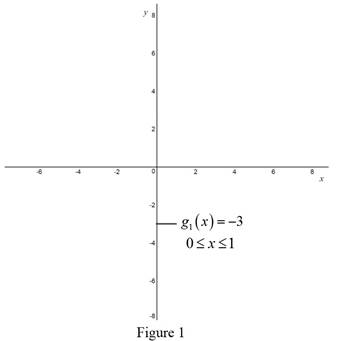Case (ii):

For 1x<2

g2(x)=x21+x24g2(x)=2x25

To sketch: Use the online graphing calculator and draw the graph of the function: g2(x)=2x2+5 as shown below in Figure 2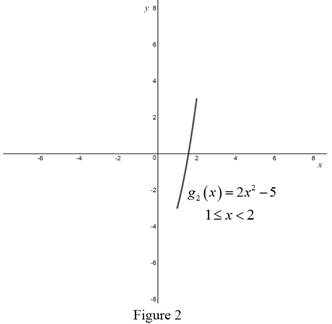Case (iii):

For x2

g3(x)=x21x2+4g3(x)=3

To sketch: Use the online graphing calculator and draw the graph of the function: g3(x)=3 as shown below in Figure 3.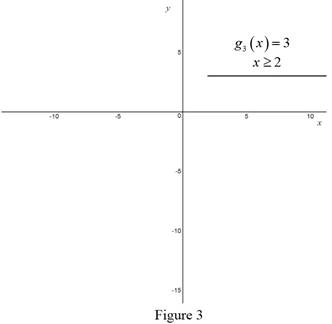Case (iv):

For 1<x0

g1(x)=x2+1x24g1(x)=3

To sketch: Use the online graphing calculator and draw the graph of the function: g1(x)=3 as shown below in Figure 4.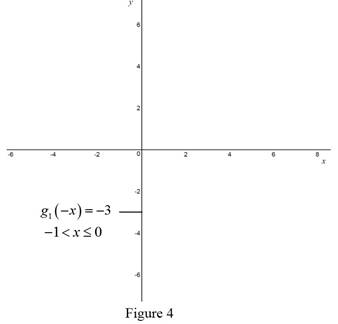Case (v):

For 2<x1

g2(x)=x21+x24 g2(x)=2x25

To sketch: Use the online graphing calculator and draw the graph of the function: g2(x)=2x2+5 as shown below in Figure 5.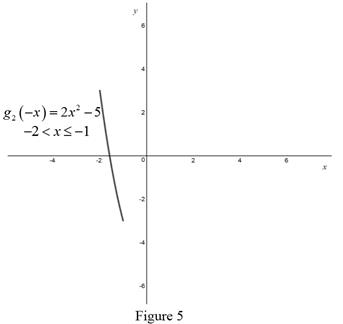Case (vi):

For x2

g3(x)=x21+x24g3(x)=3

To sketch: Use the online graphing calculator and draw the graph of the function: g3(x)=3 as shown below in Figure 6.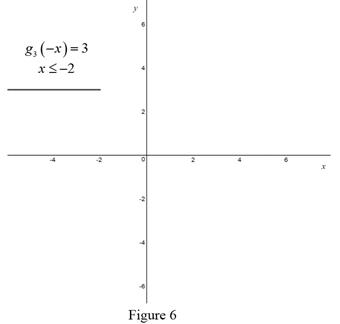Combine all the graphs in figure 1, 2,3,4,5 and 6 as shown below in Figure 7.

To sketch: Use the online graphing calculator and draw the graph of the function: g(x)=|x21||x24| as shown below in Figure 7.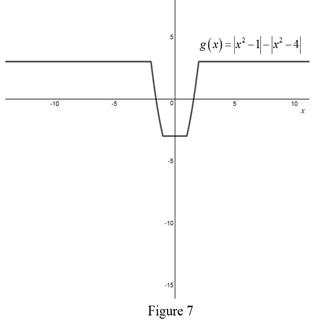### Have a homework question?

Subscribe to bartleby learn! Ask subject matter experts 30 homework questions each month. Plus, you’ll have access to millions of step-by-step textbook answers!